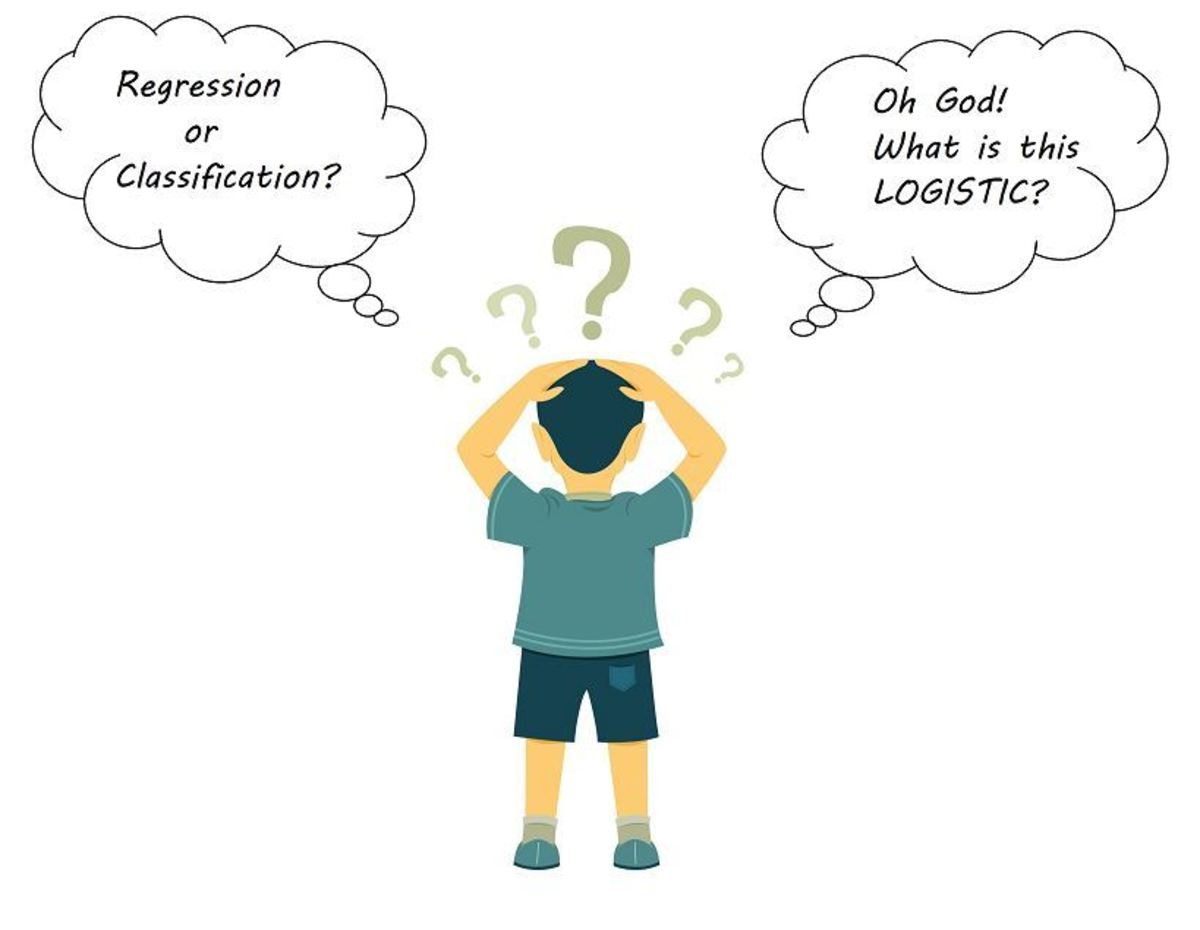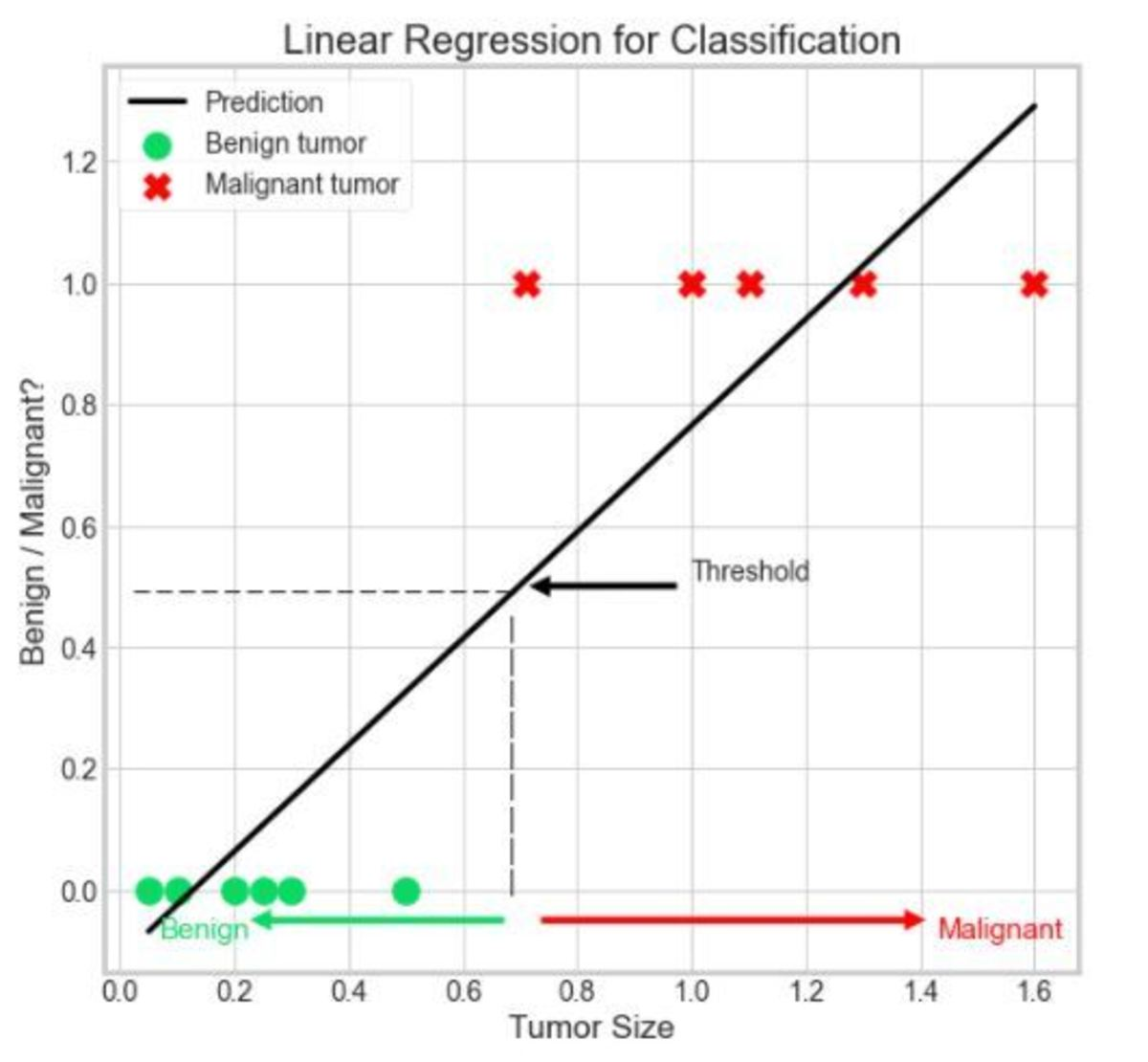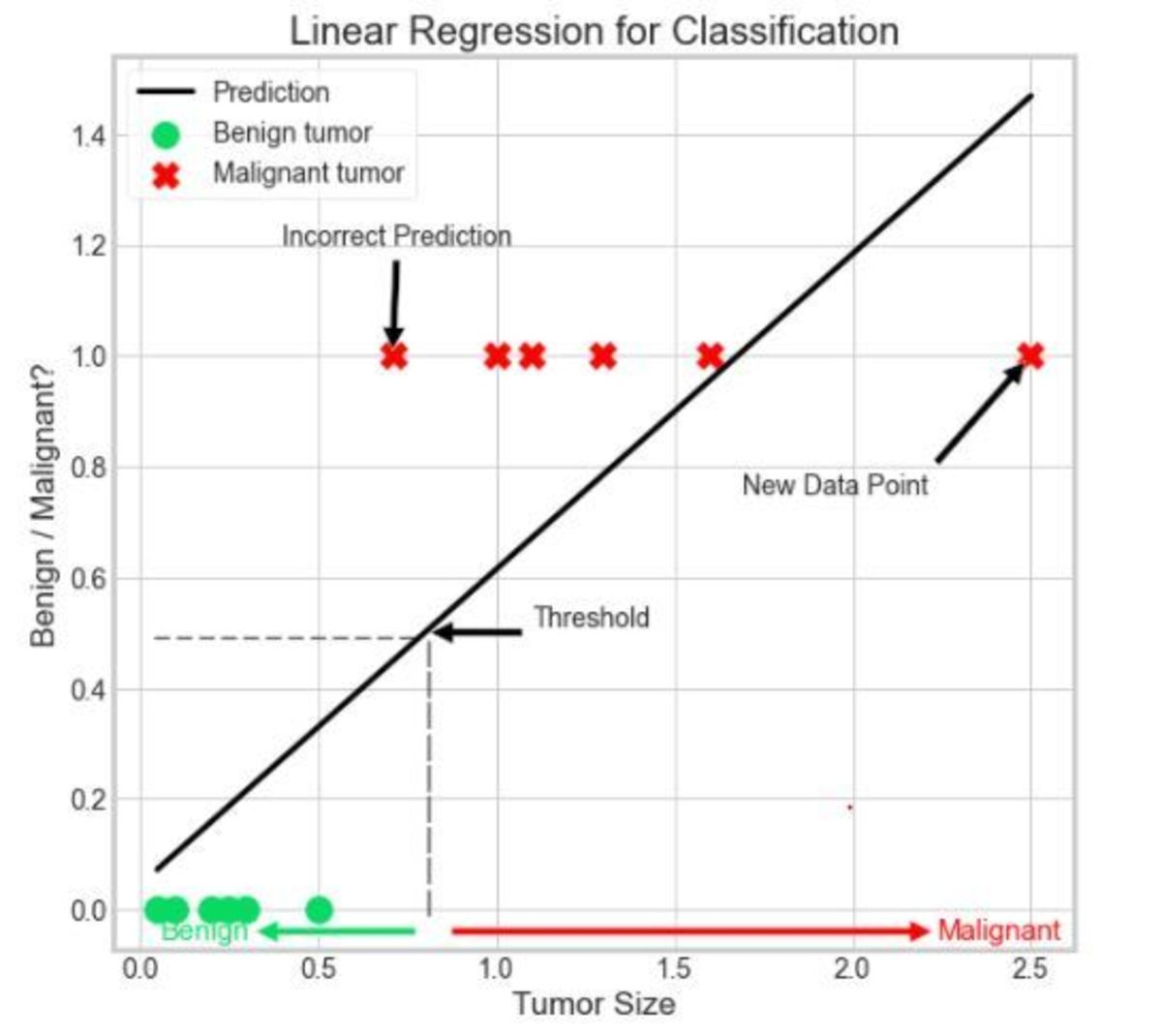# Why Is It Called "Logistic Regression" and Not "Logistic Classification"?

A computer science engineering student finding answers to science behind the tech!Why is it named "Logistic Regression"?

## Machine Learning Algorithms

Logistic Regression is one of the most popular machine learning algorithms used for binary classification, although much more complex versions are available.

One question that hits almost everyone is, "Why is it named 'Logistic Regression' if it is a classification algorithm? Why is it not 'Logistic Classification'?"

In this article, we will try to answer this question through a practical example.

## Regression vs. Classification

Let us first understand the difference between classification and regression.

Regression and classification algorithms fall under the category of supervised learning algorithms, i.e., both algorithms use labelled datasets. However, there is a fundamental difference between the two.

• Regression algorithms are used to predict continuous values such as height, weight, speed, temperature, etc.
• Classification algorithms are used to predict/ classify discrete values such as girl or boy, fraudulent or fair, spam or not spam, cold or hot, etc.

For example, if we want to predict tomorrow's temperature using a weather dataset, we use a regression algorithm. However, if we want to predict whether it is going to be hot or cold tomorrow, we use a classification algorithm.

## Why Is Linear Regression Not Suitable for Classification?

Let us try to answer the above question with the help of an example. In this section, we will try to classify benign/malignant tumors using a linear regression algorithm.

The task is to classify the tumors into Benign (0) and Malignant (1) classes respectively. Hence, it is a binary classification problem. For simplicity, we have one dependent variable—tumor size—and only a few records of the dataset.

Linear regression faces two major problems:

1. Regression outputs continuous values which cannot be treated as pure probabilistic.
2. There is an unwanted shift in the threshold value when new data points are added.

Let us discuss these problems in detail.

### Problem 1: The Output Is Not Probabilistic but ContinuousLinear Regression for Classification - 1
Scroll to Continue

Above is our linear regression model trained on our dataset. As we have already discussed, regression algorithms are used to predict continuous values, i.e., the output of linear regression is a continuous value corresponding to every input value.

But we want the output to be in the form of 1s and 0s, i.e., benign tumors and malignant tumors. Hence, we define a threshold value, 0.5 in this case.

We can predict our output as 1 (malignant) if the output of linear regression is more than or equal to the threshold value (0.5). Similarly, if the output of linear regression is less than our threshold value, we can predict our output as 0 (benign). In this way, this output can be considered as the probability of the tumor being malignant.

Wait! We just missed something. Can the probability be less than 0 or greater than 1?

Refer to the above chart, notice the output of linear regression can be both less than 0 and greater than 1. Hence, we cannot treat this output to be purely probabilistic.

### Problem 2: Unwanted Shift in the Threshold Value When New Data Points Are AddedLinear Regression for Classification - 2

Here, we have introduced a new data point in our dataset. Since linear regression tries to minimize the difference between the predicted values and actual values, when the algorithm is trained on the above dataset, it adjusts itself while taking into consideration the new data point along with other data points.

This causes a shift in the value which corresponds to the threshold value output. Notice that a few values are wrongly predicted now.

In order to solve these problems, we use logistic regression.

## Logistic Function

Logistic regression borrows "logistic" from the logistic function. The logistic function is also referred to as the Sigmoid function in the field of machine learning. (There are a number of Sigmoid functions such as hyperbolic tangent, arctangent, etc.)

This function is a bounded function as it limits/ squashes the output to a range between 0 and 1. Hence, it is also known as the squashing function. This property makes it useful to be applied in classification algorithms.

The output of the S-shaped curve is the probability of the input belonging to a certain class. For example, consider the problem of classifying a tumor as benign or malignant. If the output of this function is 0.85, then there is an 85% chance of the tumor being malignant.

Notice that the function asymptotes at 0 and 1.

## Why Is Logistic Regression Called "Logistic Regression" and Not "Logistic Classification"?

Having understood the basic concepts, we are all set to cut through the confusion.

Logistic regression borrows "regression" from the linear regression algorithm as it makes use of its hypothesis function.

In the case of linear regression, the output is the weighted sum of input variables.

hθ(x) = θ0 + θ1x1 + θ2x2 + ___ + θnxn

In logistic regression, we provide this hypothesis function as input to the logistic function.

S(x) = 1 / (1 + e-hθ(x)) = 1 / (1 + e-(θ0 + θ1x1 + θ2x2 + ___ + θnxn))

Hence, it is named as logistic regression.

## Logistic Regression Summary

• Logistic regression borrows its name from the logistic function and linear regression algorithm.
• Linear regression does not work well with classification problems.
• Logistic regression uses the logistic function which squashes the output range between 0 and 1.
• Logistic regression makes use of hypothesis function of the linear regression algorithm.

## Check Your Knowledge

For each question, choose the best answer. The answer key is below.

1. Logistic Regression is a ______ algorithm.
• Regression
• Classification
2. The output range of Logistic function is ______.
• (-ꝏ, ꝏ)
• [-1, 1]
• [0, 1]
• [-1, 0]
3. Linear Regression works very well for classification problems.
• True
• False

1. Classification
2. [0, 1]
3. False

This content is accurate and true to the best of the author’s knowledge and is not meant to substitute for formal and individualized advice from a qualified professional.

© 2021 Riya Bindra